# Data Stories

We tackle a couple of short data stories in groups. The 10 groups have been created on canvas. Cubeoctahedron Group, Disphenoid Group, Dodecahedron Group, Echidnahedron Group, Icosahedron Group, Icosidodecahedron Group, Octahedron Group, Sphenocorona Group, Tetrakishexahedron Group, Trapezohedron Group. The first project is due Friday, Februrary 5th. Get together, make a short slide show (each question one slide). Present it on Zoom while meeting, then send in the recording.

## Project 1: Primes

The first Project (PDF) deals with prime data. They are divine data, not generated by humans, but data which can be understood everywhere in the universe. If one looks at the prime data as a functions one can compute the derivative and acceleration.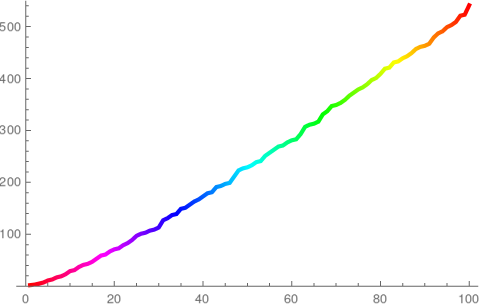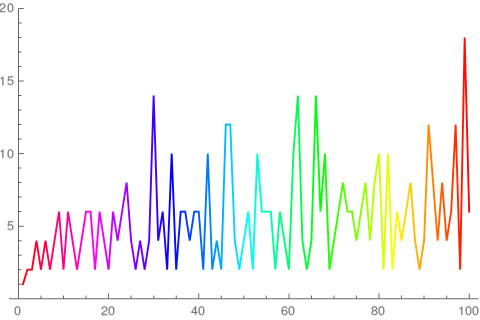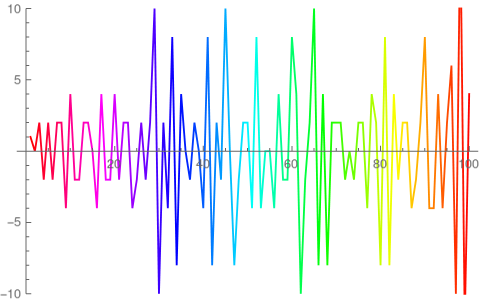Prime position Prime velocity Prime accelerationThe movie ``Contact" is based on a book of Carl Sagan. The story imagines that communication with other intelligence happens through fundamental objects like prime numbers. While biological constructs might look different in different parts of the universe, mathematics is universal.

## Project 2: Polyhedra

The second Project (PDF) deals with polyhedra. They are beautiful too and related to calculus. Every network G defines a function fG(x). The problem of computing this function is known to be the hardest problem in computer science: it is NP complete. We learn here how to compute the function from local data. It is interesting that like primes, which were studied initially for purely mathematical reasons and which then exploded to the most important encryption and key exchange technology, also polyhedra were studied originally since Plato for their purity but are now part of a theory of networks which is used in tacking modern problems of communication, computation, logistics and are at the heart of complexity theory: if we were able to compute fG(x) effectively, we could solve essentially all hard problems in computer science as it would settle the P versus NP problem.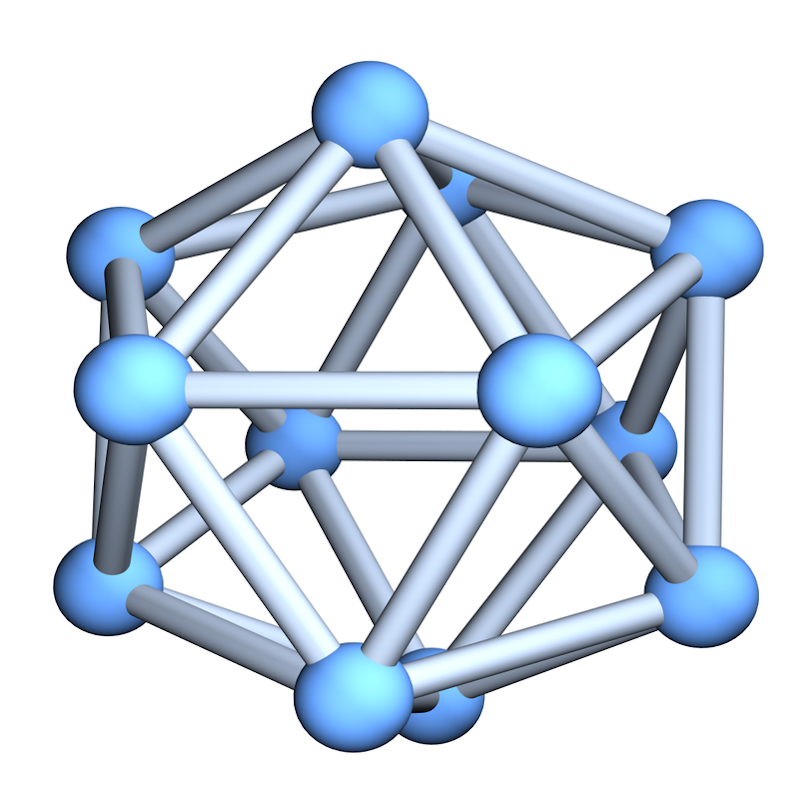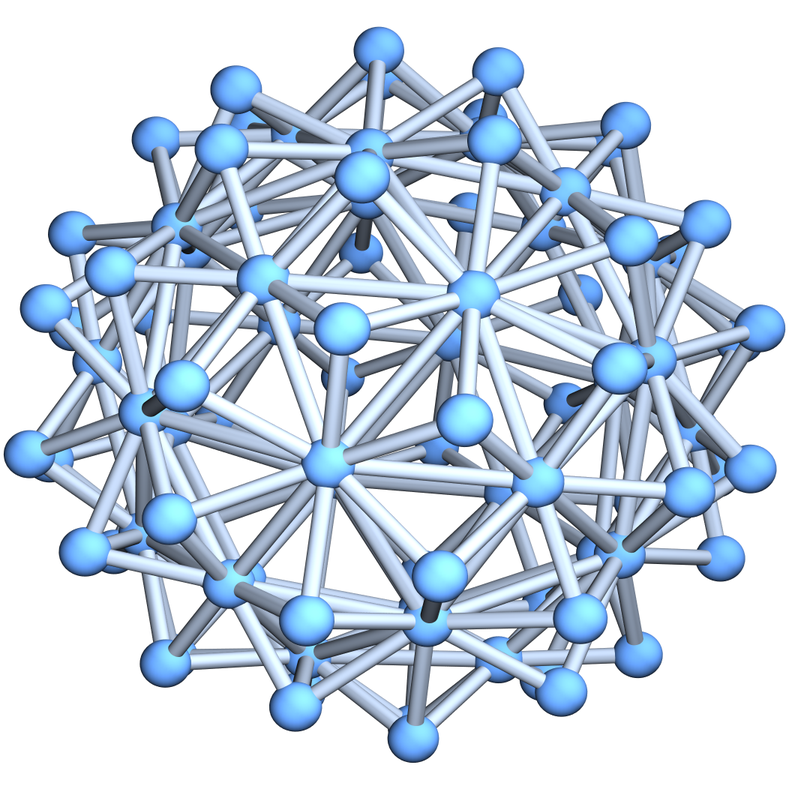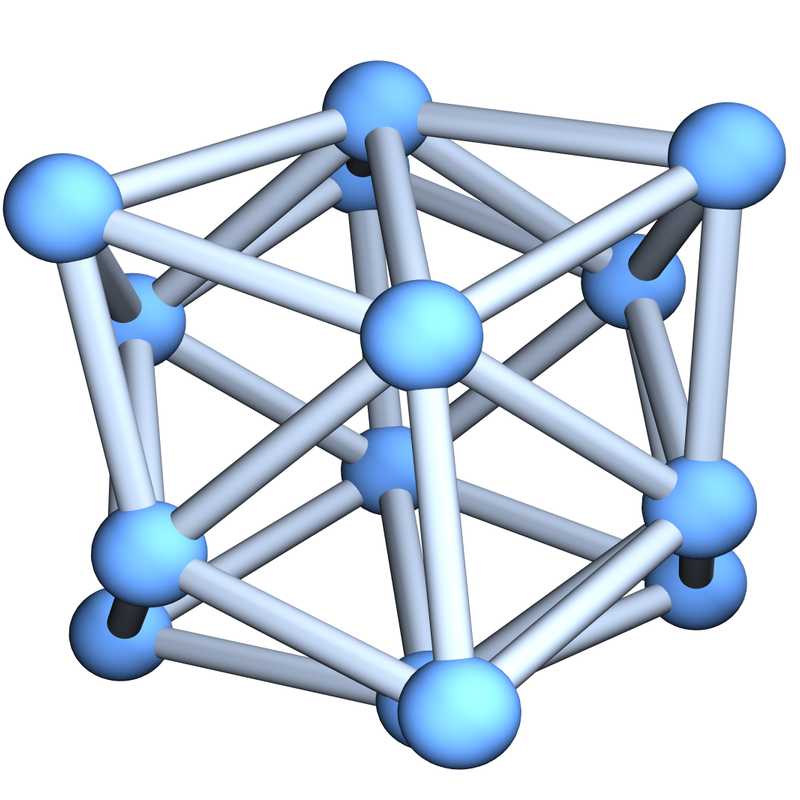Icosahedron Echidnahedron Tetraxishexahedron
The movie ``Travelling Salesman" appeared in 2012. It is a stage play similarly as the Swiss play ``The physicists" written by Dürrenmatt. [ As Singh once pointed out, WW1 was the war of the Chemists (Gas), WW2 was the war of the Physicists (A-Bomb) WW3 most likely will be the war of Mathematicians (Communication).] In the Travelling Salemen movie, the conversation builds on a scenario, in which the NP problem was solved affirmatively. Nobody who works in the field however believes that P=NP holds. The goal is to prove N and NP are different. Like the scenario that extraterrestial life exists which will be able to get in contact with us, the scenario N=NP makes good Holliwood stuff.

## Data 3: Chaos

 The third Project (PDF) deals with the iteration of maps on the interval [0,1]. We wrote this Desmos applet. It allows you to see that f(x)=4x(1-x) and g(x)=4x-4x2 produces different results when we apply the function several times. This always happens if we have sensitive dependence on initial conditions, a term which also goes under the name ``chaos". We see that when starting with x0=0.84, then f60(x0) = 0.93295901 but g60(x0) = 0.45183101. What about changing the tool? In Mathematica ``` Mathematica 12.1.1 Kernel for Linux x86 (64-bit) Copyright 1988-2020 Wolfram Research, Inc. In:= Last[NestList[Function[x,4x(1-x)],0.84,60]] Out= 0.658493 In:= Last[NestList[Function[x,4x-4x^2],0.84,60]] Out= 0.86512 ``` gives even completely different numbers. This is not a problem of the calculators. No, it is in the nature of things that processes can exhibit sensitive dependence on initial conditions. This is chaos.
The movie ``Jurassic Park" makes the topic of chaos a theme.

## Data 4: Monte Carlo

The fourth and last project deals with Monte Carlo computations. Here is the project (PDF) . Also this project is short. There are only 2 problems. The first is to use the digits of pi to integrate a function. The second is to estimate the area of the Mandelbrot set using Monte Carlo integration.

Both of the problems are related to difficult mathematical unsolved problems: we do not know whether the digits of pi are random. We believe they are so. What does that mean? There is a nice theory called ergodic theory which makes this clear; one can use the digits of pi and all the shifted versions to get a compact space called the ``hull". Shifting the digits produces a continuous map on this space. The mathematical conjecture is that this dynamical system is a Bernoulli shift with uniform weights. This would justify that the pi is what one calls ``normal" and that all statistical tests for random numbers are satisfied. The digits would come all with the same frequency for example. Especially, we could use the digits of pi to generate random numbers. The second problem is to compute the area of the Mandelbrot set. To appreciate this problem, one must go back 2500 years which was a time, when the volume of the sphere was not known. It turned out that the volume of the sphere can be computed and Archimedes has shown us how to do that. As for the area of the mandelbrot set, one would like to know whether the number is expressible using known constants. Is it rational? Is it algebraic (a root of a polynomial with integer coefficients) or expressible using constants like pi, e, sqrt(2) etc? We have currently not even the slightest clue how to attack this problem. Yes, one can express it as a limit by approximating the Mandelbrot set with smooth finite approximations but this does not tell anything about the limit. Also here there is a precedent: the sum 1+1/4+1/9 + 1/16 + 1/25 + ... was at the time of Euler not known. It was a challenge called the Basel problem. It was Euler who realized that it is pi^2/6. Before Euler solved this, one did not now whether there is a simple solution. It could have been inaccessible like 1+1/8+1/27+1/64 + ..., the sum of cube reciprocals which is called the Apery's constant. That number is up to now not known whether it is algebraic or not. As for the Mandelbrot set, we can challenge ourselves to get better and better numerical approximations of the number.

In the first part, we use the digits of pi as a number generator. Lets illustrate this with taking 3 digit blocks. Here are 60 digits of pi
```3.14159265358979323846264338327950288419716939937510582097494
```
We can produce random numbers by building blocks of 3 to get the sequence
``` 141, 592, 653, 589, 793, 238, 462, 643, 383, 279,
502, 884, 197, 169, 399, 375, 105, 820, 974, 944
```
Now we get random numbers by dividing by 1000
```x1= 0.141, x2= 0.592 , x3=0.653   etc
```
Now, we can integrate a function like f(x)=x3 numerically by building the sum (∑k=120 (xk)^3)/20. In this case the sum is 0.2338 which is still a bit off from the 0.25 we want to have. But when using more digits this converges. For 600 digits, we get 0.244244, with 1000 digits we get 0.25467. Here is what we get depending on the number of digits. The k'th entry in this block uses 3k digits of pi so that we have k numbers xk.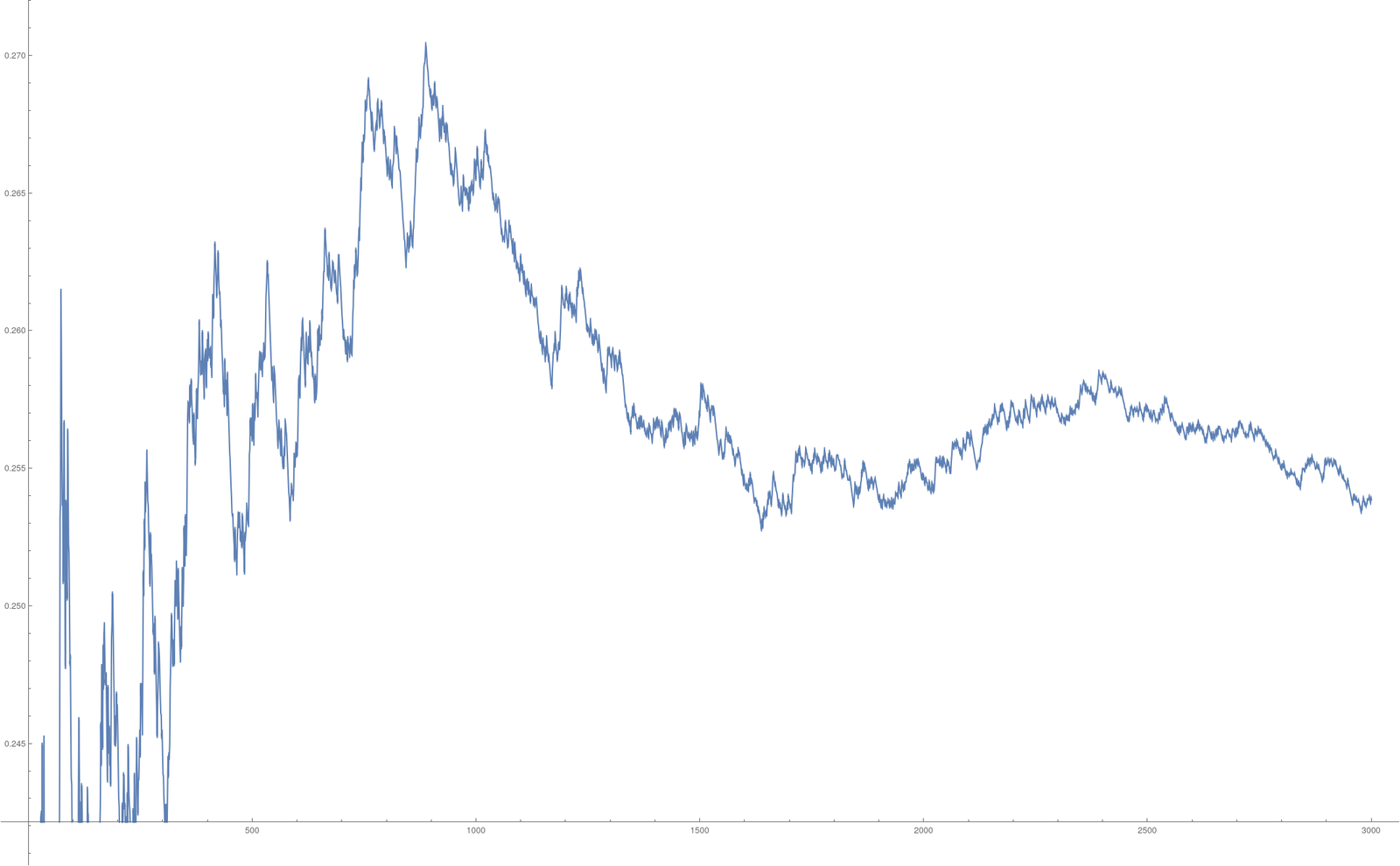In the next picture we go to 30000 digits and also plot the data xk which appear pretty random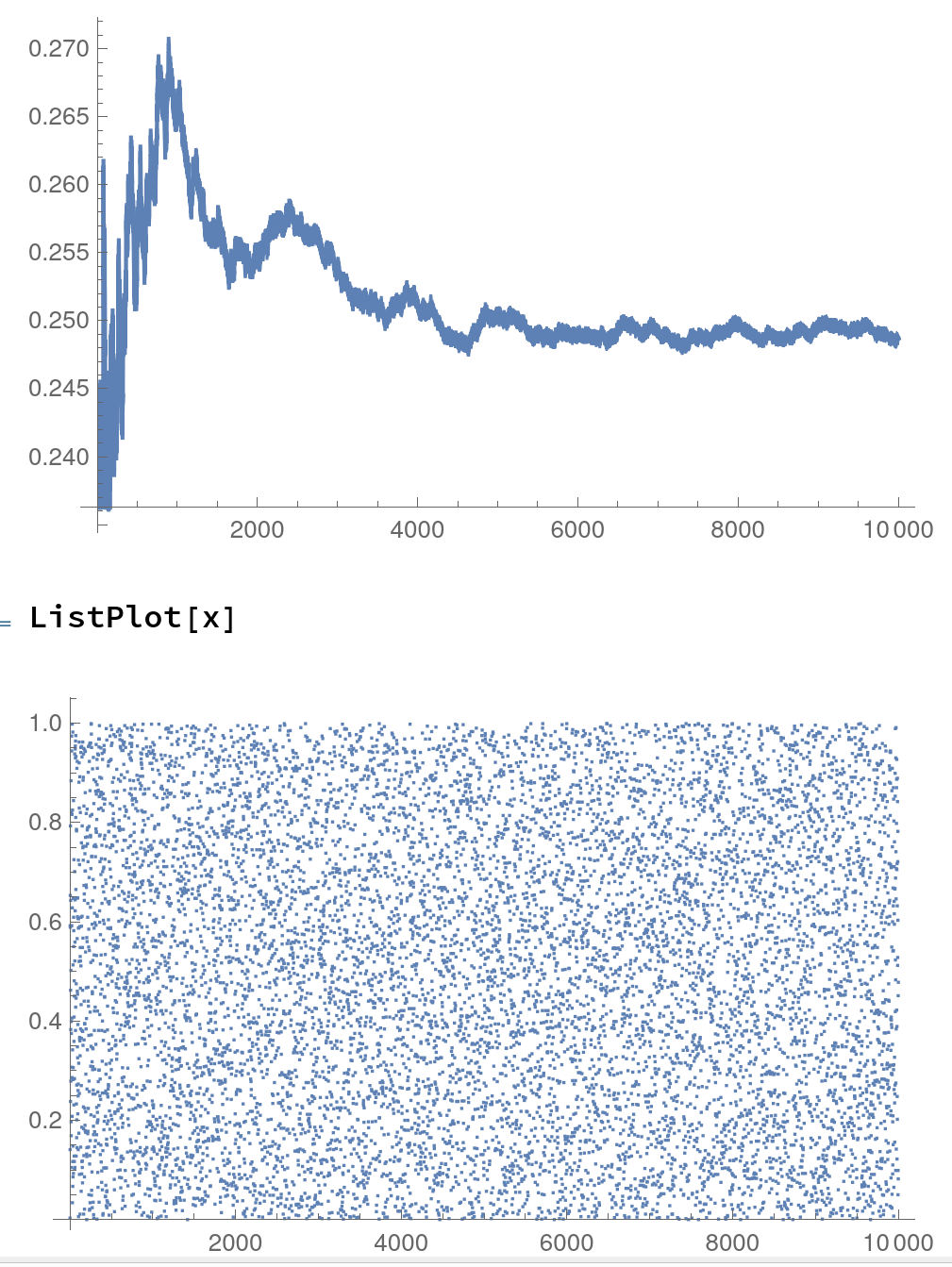In the second part, we shoot n randomly points into the box A given by { (x,y) with -2 ≤ x &le 1 and -1.5 ≤ y &le 1.5} and see how many times m we hit. The number (m/n)*box area = 9m/n is then an approximation of the Area of the Mandelbrot set. The Riemann integral would be completely inadequate here as the boundary of the Mandelbrot set is very complicated. Its dimension is even 2 and not 1 as for a curve (a result from 1991.

Here is the online python interpreter and here is the python code. Try to tune the parameters to get more accurate results!

The area of the mandelbrot set appeared previously in the following Project of Math S 21a of Fall 2019. There is some more information there.
The following picture of the Mandelbrot set was produced with the Ray tracer Povray. You find the source code of a Povray program which produces a movie on this page. The program is so small that it could be tweeted. Here is the tweet and the movie.